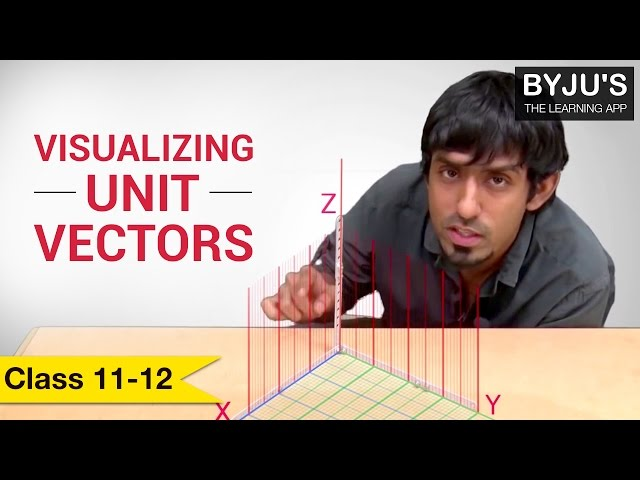# Vector Algebra Class 12 Notes - Chapter 10

## Position of a Vector

If we are provided with a point Q(x,y,z) and

$$\begin{array}{l}\overline{OQ}(=\underset{r}{\rightarrow})=x\widehat{i}+y\widehat{j}+z\widehat{k}\end{array}$$
and the magnitude is given by
$$\begin{array}{l}\sqrt{x^{2}+y^{2}+z^{2}}\end{array}$$
. The direction ratios for a vector is its scalar components and is responsible for its projections along the respective axes.

## CBSE Class 12 Maths Notes Chapter 10 Vector Algebra – Related Links### The relation between magnitude, direction ratios, direction cosines of a vector

If a vector has been given with dimensions such as magnitude(p), direction ratios (x,y,z) and direction cosines (l,m,n) then the relation between them is:

l=x/p, m=y/p,n=z/p

The order taken for the vector sum of the three sides of the triangle is

$$\begin{array}{l}\underset{0}{\rightarrow}\end{array}$$

And the vector sum of coinitial vectors is the diagonal of the parallelogram which has the vectors as its adjacent sides.

When multiplying a vector by a scalar λ, the magnitude of the vector changes by the multiple |λ|, and the direction remains same (or makes it opposite) according to as the value of λ is positive (or negative).

### Position Vector of a Point R on a Line Segment

A line segment joining two points such as P having a position vector of

$$\begin{array}{l}\underset{a}{\rightarrow}\end{array}$$
and Q having a position vector of
$$\begin{array}{l}\underset{b}{\rightarrow}\end{array}$$
is divided by a point R by the ratio m;n then,

• The internal ratio is given by
$$\begin{array}{l}n\underset{a}{\rightarrow}+m\underset{b}{\rightarrow}/m+n\end{array}$$
• The external ratio is given by

$$\begin{array}{l}m\underset{b}{\rightarrow}-n\underset{a}{\rightarrow}/m-n\end{array}$$

The scalar product of two position vectors

$$\begin{array}{l}\underset{a}{\rightarrow}\end{array}$$
and
$$\begin{array}{l}\underset{b}{\rightarrow}\end{array}$$
and subtending an angle
$$\begin{array}{l}\theta\end{array}$$
is represented by
$$\begin{array}{l}\underset{a}{\rightarrow}\end{array}$$
.
$$\begin{array}{l}\underset{b}{\rightarrow}\end{array}$$
=
$$\begin{array}{l}\left | \underset{a}{\rightarrow} \right |\left | \underset{b}{\rightarrow} \right |cos\theta\end{array}$$

We can find the value of

$$\begin{array}{l}\theta\end{array}$$
from the above equation.

### Important Questions

1. Find the ratio in which B divides AC and also show that the points A(2, – 3, – 9), B(6, 0, –1) and C(10, 4, 6) are not collinear
2. Determine the girl’s displacement from her initial point of departure if she walks 5 km towards west, then she walks 4 km in a direction 40° east of north and stops.
3. Draw a unit vector in the XY-plane, making an angle of 30° with the positive direction of x-axis
4. Find the area of the triangle with vertices P(2, 3, 4), Q(3, 4, 6) and R(2, 6, 7).
5. Show that the points P(1, 2, 7), Q(2, 6, 3) and R(3, 10, –1) are collinear

## Frequently asked Questions on CBSE Class12 Maths Notes Chapter 10: Vector Algebra

### What is a ‘Constant’?

Constant is a quantity which has a fixed value.

### What are ‘Like and Unlike’ terms?

In algebra, like terms are terms that have the same variables and powers. Unlike terms are two or more terms that are not like terms, i.e. they do not have the same variables or powers.

### What is a ‘Vector’ quantity?

Any quantity that has both magnitude and direction but not position is known as a Vector quanity.